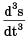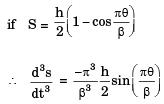Courses

# Past Year Questions: Cams And Follower

## 6 Questions MCQ Test Engineering Mechanics | Past Year Questions: Cams And Follower

Description
This mock test of Past Year Questions: Cams And Follower for Mechanical Engineering helps you for every Mechanical Engineering entrance exam. This contains 6 Multiple Choice Questions for Mechanical Engineering Past Year Questions: Cams And Follower (mcq) to study with solutions a complete question bank. The solved questions answers in this Past Year Questions: Cams And Follower quiz give you a good mix of easy questions and tough questions. Mechanical Engineering students definitely take this Past Year Questions: Cams And Follower exercise for a better result in the exam. You can find other Past Year Questions: Cams And Follower extra questions, long questions & short questions for Mechanical Engineering on EduRev as well by searching above.
QUESTION: 1

### In a cam-follower mechanism, the follower needs to rise through 20 mm during 60° of cam rotation, the first 30° with a constant acceleration and then with a deceleration of the same magnitude. The initial and final speeds of the follower are zero. The cam rotates at uniform speed of 300 rpm. The maximum speed of the follower is 

Solution: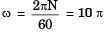Time taken to move 30°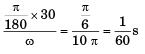During this period follower move 20 mm = .02 m and  u = 0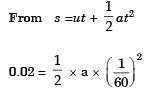a =144 m/sec2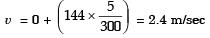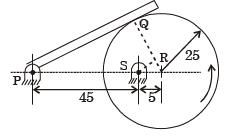QUESTION: 2

### In the mechanism given below, if the angular velocity of the eccentric circular disc is 1 rad/s, the angular velocity (rad/s) of the follower link for the instant shown in the figure is [Note : All dimensions are in mm.]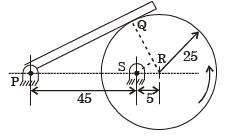Solution:

ΔPQR ~ ΔSRO
∴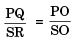...(1)
(PO)2 = (PQ)2 + (OQ)2
∴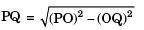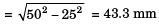From (1),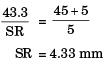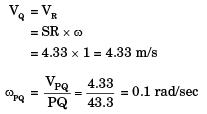QUESTION: 3

### Consider a rotating disk cam and a translating roller follower with zero offset. Which one of the following pitch curves, parameterized by t, lying in the interval 0 to 2π, is associated with the maximum translation of the follower during one full rotation of the cam rotating about the center at (x, y) = (0, 0)? 

Solution:

From all the four options the maximum amplitudes is in point ‘C’ as t = 0.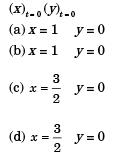Option ‘c’ has maximum net amplitude.

QUESTION: 4

In a cam-follower, the follower rises by h as the cam rotates by d (radians) at constant angular velocity w (radians/s). The follower is uniformly accelerating during the first half of the rise period and it is uniformly decelerating in the later half of the rise period. Assuming that the magnitudes of the acceleration and deceleration are same, the maximum velocity of the follower is



Solution:

Here, out stroke angle
θo = δ
and stroke length = h
Angular velocity = ω
V = u + at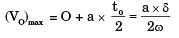...(1)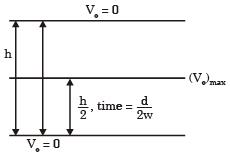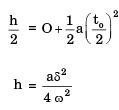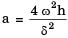...(2)
From (1) & (2), we get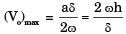QUESTION: 5

A flat -faced follower is driven using a circular eccentric can rotating at a constant angular velocity ω. At time t = 0, the vertical position of the follower is y(0) = 0, and the system is in the configuration shown below.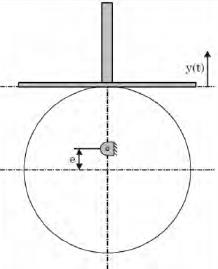The vertical position of the follower face, y(t) is given by



Solution: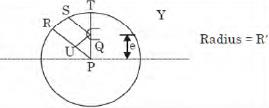As the follower starts moving, the displacement Y can be shown as marked in the diagram
Y = QS – QT
= RU – QT (∵ QS||RU)
= (PR – PU ) – QT ...(i )
PR = R'
⇒ PU = e cos θ
QT = (R' - e) from geometry
Now, putting the above values in equation (i)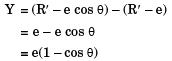⇒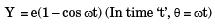QUESTION: 6

In a cam design, the rise motion is given by a Simple Harmonic Motion (SHM)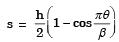where h is total rise, θ is camshaft angle, β is the total angle of the rise interval. The jerk is given by



Solution:

The jerk is given by